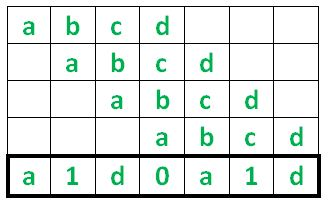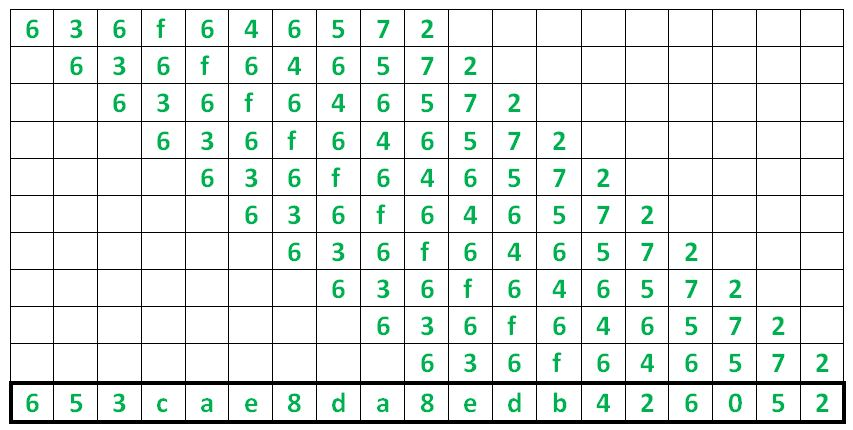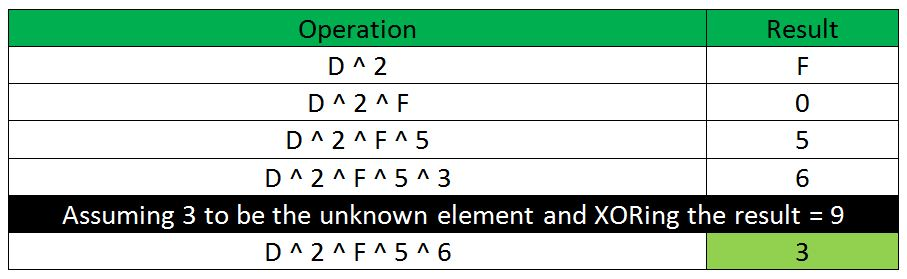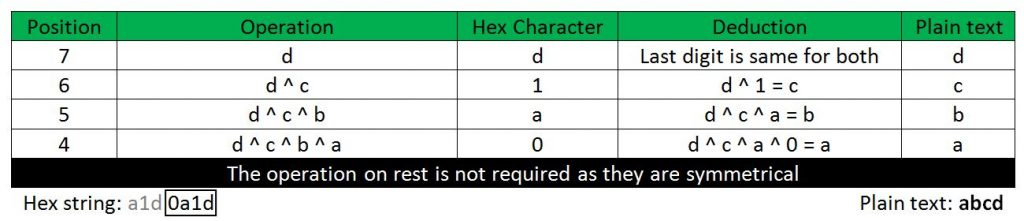# XOR Encryption by Shifting Plaintext

Here is a cipher algorithm, based on hexadecimal strings that is implemented by XORing the given plaintext, N number of times where N is its length. But, the catch is that every next XOR operation is done after shifting the consecutive plain text entry to the right. A sample operation is shown below :Suppose the password is ‘abcd’ then the hexadecimal text is calculated as a1d0a1d by XORing the password with itself N times i.e. 4 times in this case.

Similarly if the password is ‘636f646572’, thenSo, the problem statement is to create a decryption algorithm (in any programming language) and deduce the plain text from the given hexadecimal string.

Examples :

```Input : a1d0a1d
Output : abcd
abcd once coded will return a1d0a1d

Input : 653cae8da8edb426052
Output : 636f646572
```

Approach : The key ingredient in encrypting and decrypting is in the properties of XOR. XOR is a bitwise operation where the result is 0 if the two possible inputs are same but 1 when the inputs are different. The XOR table is given below for reference :

Inputs Outputs
X Y Z
0 0 0
0 1 1
1 0 1
1 1 0

An important and useful property of XOR that is widely popular in cryptography is that in case of multiple XORing of numbers (say M numbers), if we know only the M – 1 numbers (one is unknown) along with the XOR result then, we can easily calculate the missing number by XORing the known numbers and the XOR result. This property is discussed with the following hexadecimal numbers :We shall be using the above listed property the most in course of this problem. Now, if we look at the encryption diagram of ‘abcd’ at the base it is just the repeated XORing of the digits. The rightmost digit is d and the rightmost digit of the ‘abcd’ is d as well so the last digit of both plaintext and hexstring is the same. The next digit is 1 which is calculated by XORing the second right digit of abcd and the previous digit i.e. 1 = d ^ c using the property we know the plain text digit can be deduced as d ^ 1 = c. Similarly, the next digit is a which is found by d ^ c ^ b = a. We only need to do this till the half of the hex string as the rest is symmetrical so they are not required.Below is the implementation of above approach :

 `# Implementation in Python 3 ` ` `  `# Hex String variable ` `hex_s ``=` `'653cae8da8edb426052'` ` `  `# Plain text variable ` `plain ``=` `'' ` ` `  `# variable to store the XOR ` `# of previous digits ` `x ``=` `0` ` `  `l ``=` `len``(hex_s) ` ` `  `# Loop for loop from the end to ` `# the mid section of the string ` `for` `i ``in` `range``(l ``-` `1``, ``int``(l ``/` `2``) ``-` `1``, ``-``1``): ` `     `  `    ``# calculation of the plaintext digit ` `    ``y ``=` `x^``int``(hex_s[i], ``16``) ` `     `  `    ``# calculation of XOR chain ` `    ``x ``=` `x^y ` `    ``plain ``=` `hex``(y)[``-``1``] ``+` `plain ` `     `  `print``(plain) `

Output:

```636f646572
```

Don’t stop now and take your learning to the next level. Learn all the important concepts of Data Structures and Algorithms with the help of the most trusted course: DSA Self Paced. Become industry ready at a student-friendly price.

My Personal Notes arrow_drop_upCheck out this Author's contributed articles.

If you like GeeksforGeeks and would like to contribute, you can also write an article using contribute.geeksforgeeks.org or mail your article to contribute@geeksforgeeks.org. See your article appearing on the GeeksforGeeks main page and help other Geeks.

Please Improve this article if you find anything incorrect by clicking on the "Improve Article" button below.